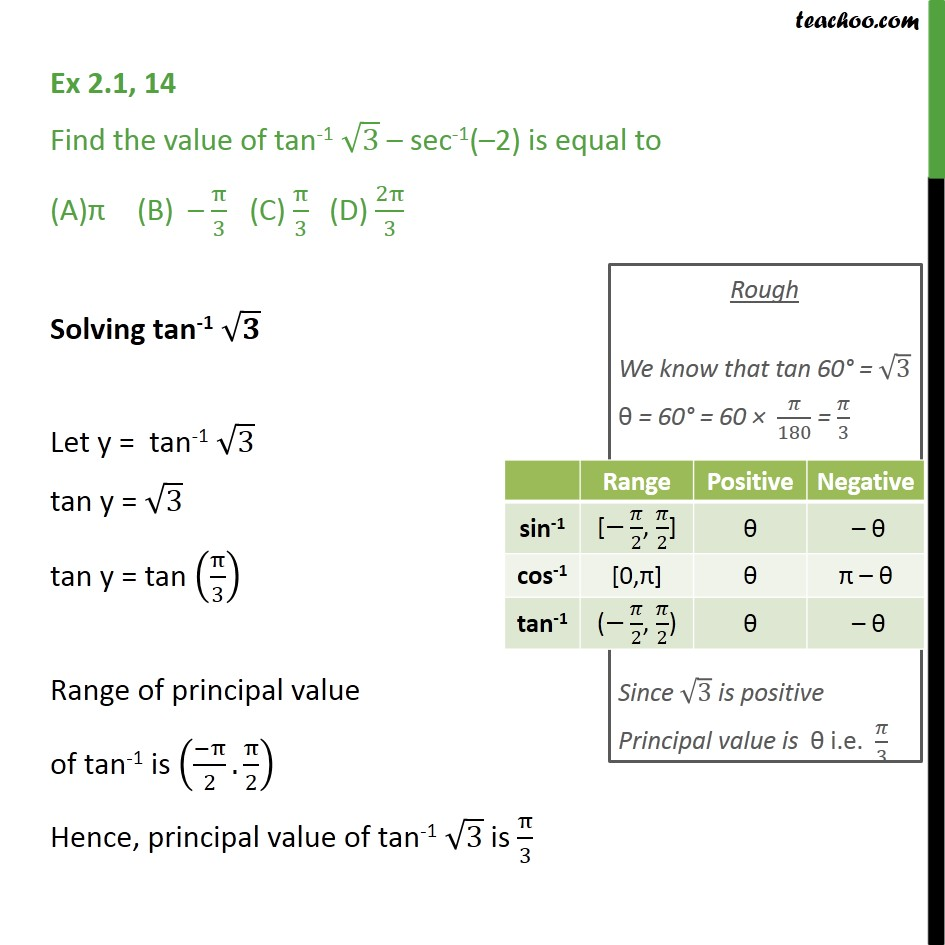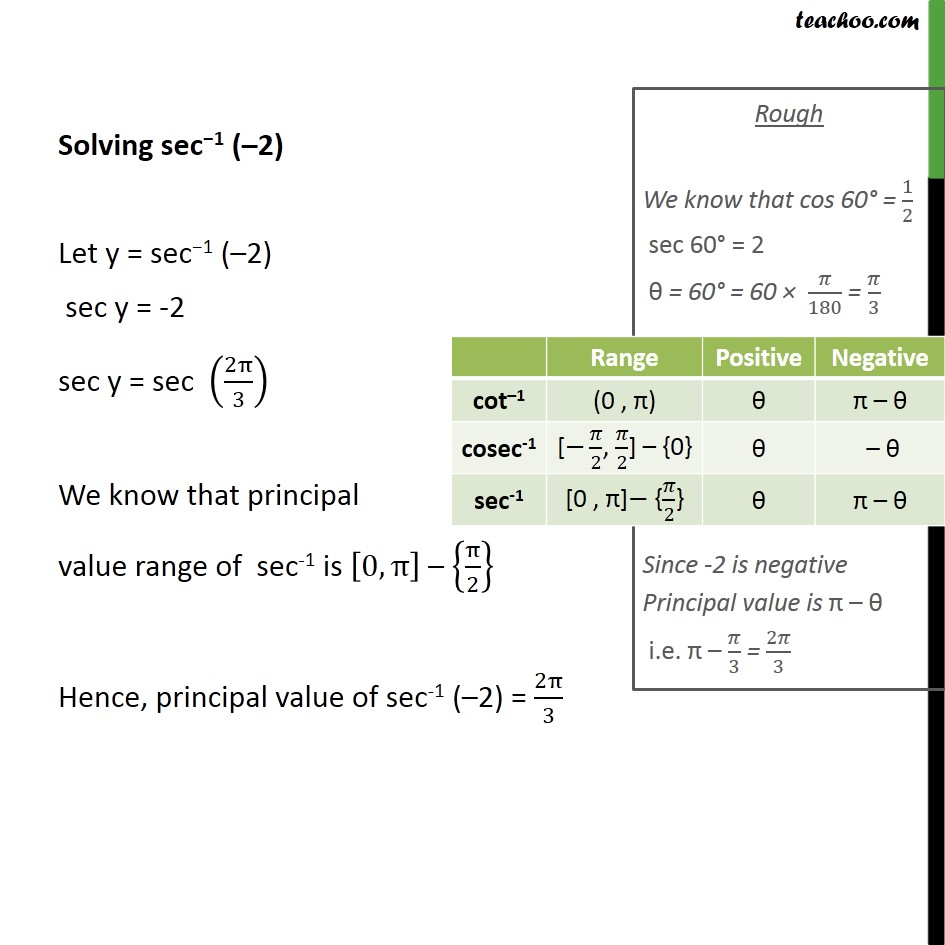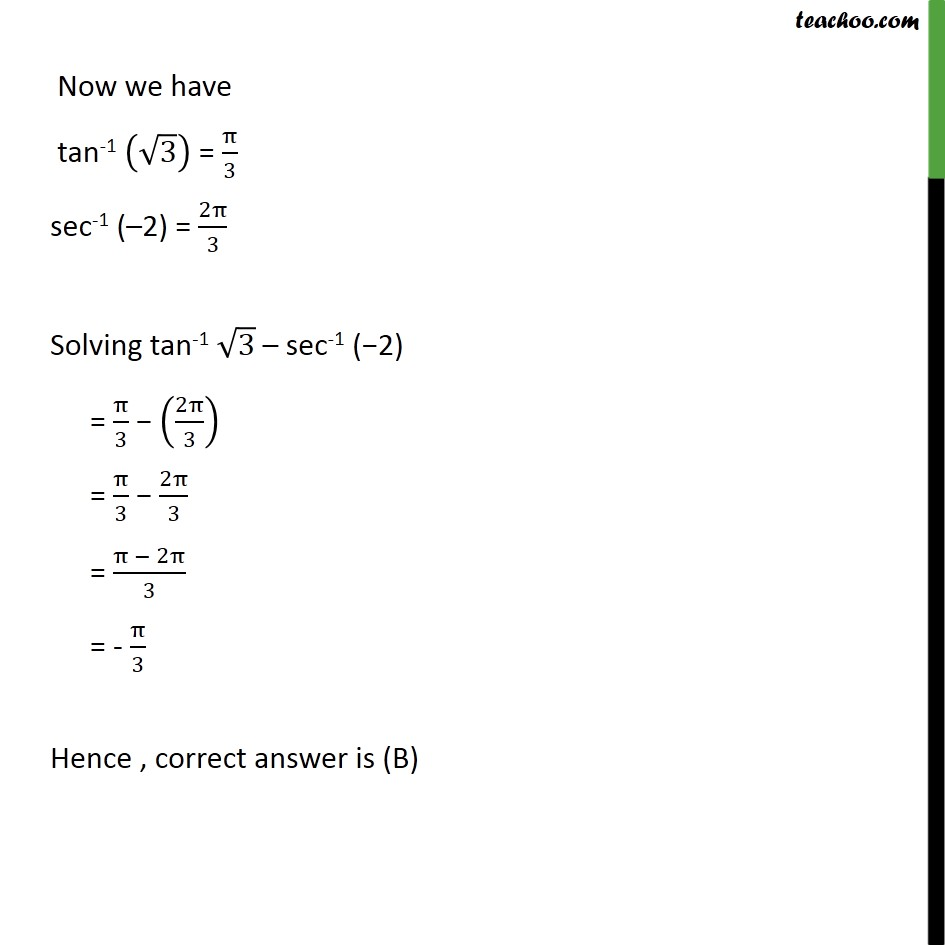1. Class 12
2. Important Question for exams Class 12
3. Chapter 2 Class 12 Inverse Trigonometric Functions

Transcript

Ex 2.1, 14 Find the value of tan-1 √3 – sec-1(–2) is equal to π (B) – π/3 (C) π/3 (D) 2π/3 Solving tan-1 √𝟑 Let y = tan-1 √3 tan y = √3 tan y = tan (π/3) Range of principal value of tan-1 is ((−π)/2.π/2) Hence, principal value of tan-1 √3 is π/3 Solving sec−1 (–2) Let y = sec−1 (–2) sec y = -2 sec y = sec (2π/3) We know that principal value range of sec-1 is [0,π] – {π/2} Hence, principal value of sec-1 (–2) = 2π/3 Now we have tan-1 (√3) = π/3 sec-1 (–2) = 2π/3 Solving tan-1 √3 – sec-1 (−2) = π/3 − (2π/3) = π/3 − 2π/3 = (π − 2π)/3 = - π/3 Hence , correct answer is (B)

Chapter 2 Class 12 Inverse Trigonometric Functions

Class 12
Important Question for exams Class 12